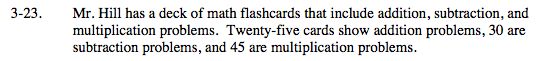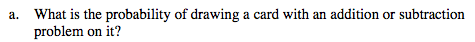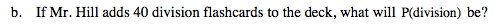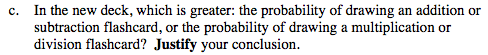### Home > MC2 > Chapter 3 > Lesson 3.1.2 > Problem3-23

3-23.$\text{P(additional problem) }= \frac{25}{100}$

$\text{P(subtraction problem) }= \frac{30}{100}$

Since you know how to get the probability of drawing an addition or a subtraction problem separately, how could you get the probability of drawing either an addition or subtraction problem in one draw?

Try adding the separate probabilities together. Does this make sense? Why?If the 40 division flashcards are added, how many division problems would there be in the deck?

How many total cards are in the deck now?
Remember, 40 cards were just added so the total amount of cards is larger.

$\frac{40}{140} = \frac{2}{7}$Out of the new deck with the 40 added division cards, is it more likely to draw either an addition or subtraction card or to draw either a multiplication or division card?

Drawing a multiplication or division card is more likely. Why is this the answer?